# Shuffling an Array in JavaScript

by kirupa   |   filed under Arrays

Use the Fisher-Yates / Knuth approach to quickly shuffle the contents of an array in JavaScript.

Let's say we have an array whose contents are the numbers 1 through 9 stored sequentially as visualized below:There will be times when you want to kick things up a few notches. One way of doing this is by shuffling our array's contents to end up with something like the following: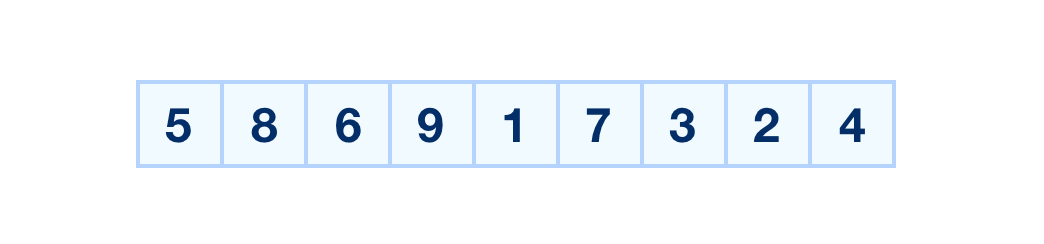As you will find out, you will frequently find yourself needing to shuffle the contents of an array. To prepare you for such times, we are going to look at and learn about an efficient way to make all these array shuffling shenanigans happen.

Onwards!

#### OMG! An Arrays Book Written by Kirupa?!!

To kick your array skills into the stratosphere, everything you need to be an arrays expert is available in both paperback and digital editions.

## Code for Shuffling an Array

The approach we will use for shuffling the contents of an array is something Fisher-Yates devised and Don Knuth popularized. The general approach they came up with can be seen in code form below:

``````Array.prototype.shuffle = function () {
let input = this;

for (let i = input.length - 1; i >= 0; i--) {

let randomIndex = Math.floor(Math.random() * (i + 1));
let itemAtIndex = input[randomIndex];

input[randomIndex] = input[i];
input[i] = itemAtIndex;
}
return input;
}

let tempArray = [1, 2, 3, 4, 5, 6, 7, 8, 9];
tempArray.shuffle();

// and the result is...
``````

The shuffle function is responsible for shuffling the contents of our array. It is declared as a prototype on the built-in Array object, so we can use it on any array we create as shown below:

``````let tempArray = [1, 2, 3, 4, 5, 6, 7, 8, 9];
tempArray.shuffle();
console.log(tempArray);``````

If all you wanted was the code to shuffle the contents of an array in JavaScript, you can pretty much stop here. If you wanted to be really cool (or cooler!) and learn a bit more about how the shuffling actually works, you should read on 🤖

## How the Shuffling Works

The best way to understand what the code does is to first take a step back and learn (using words and pictures) more about how our shuffling actually works. We will start at the top by looking at the array we kicked everything off with:The first thing we do is start at the end of our array by selecting our last item, the one containing the 9: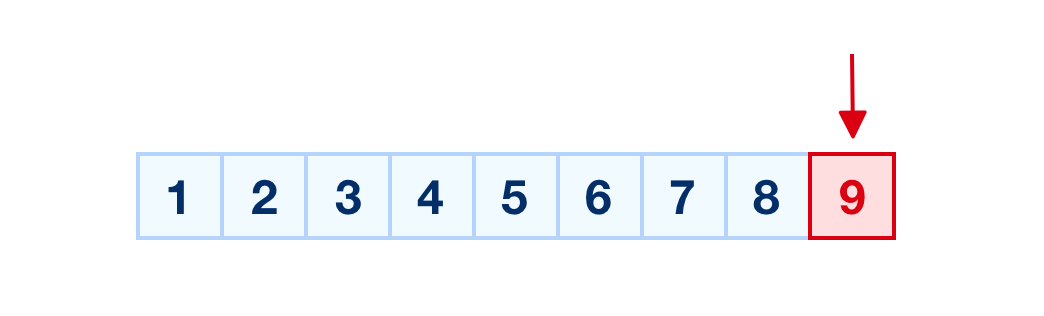What we are going to do is swap the contents of our currently selected item with another item randomly selected from our array. The range of items we can randomly pick from are the selected item itself and all items that precede it: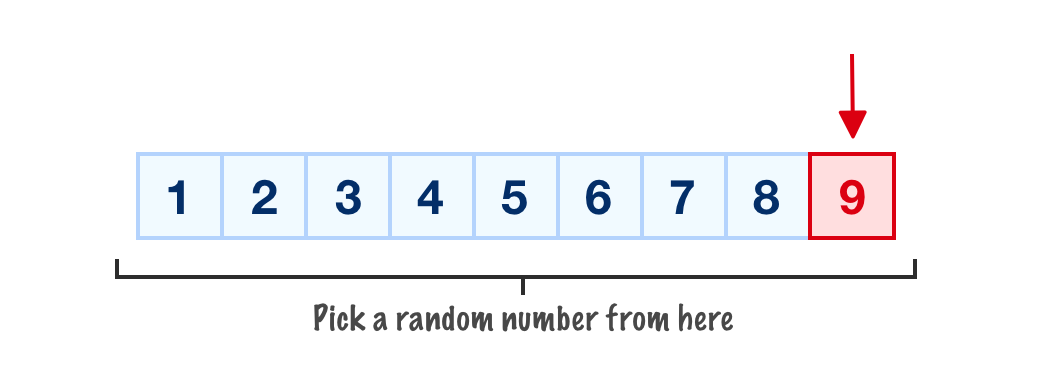Since this is our first run, every item in our array is fair game for being chosen. Let's say that the random item we pick is the one containing the 4: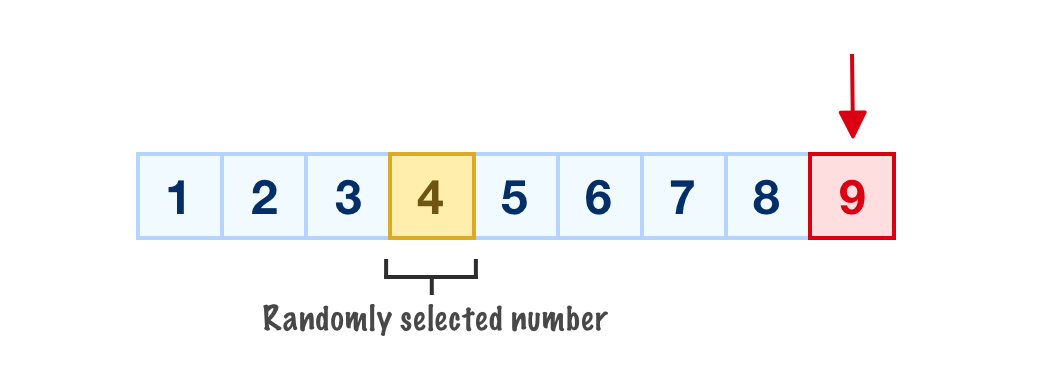Once the random number has been picked, we swap the contents of our selected item with the randomly selected item. This would mean the 9 and the 4 swap places: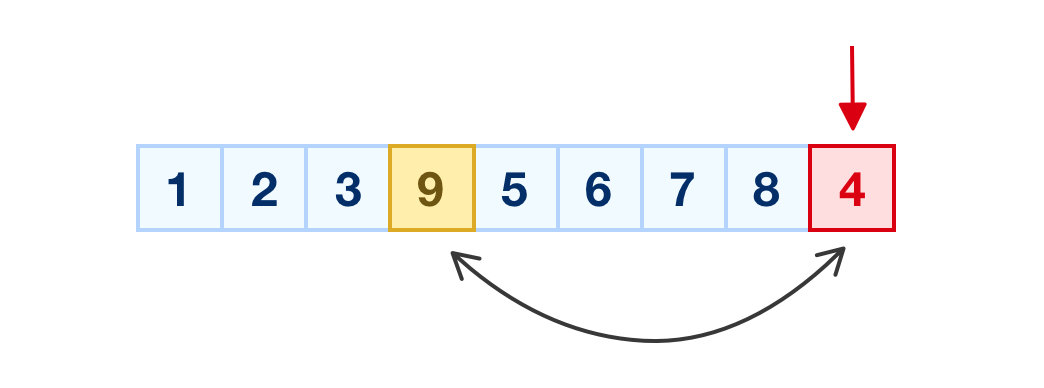With the swap completed, we are done with our last item. We are also done with the first run of our shuffling approach. We still have many more items that need to be shuffled, so we traverse through our array backwards and now pick our second-to-last item: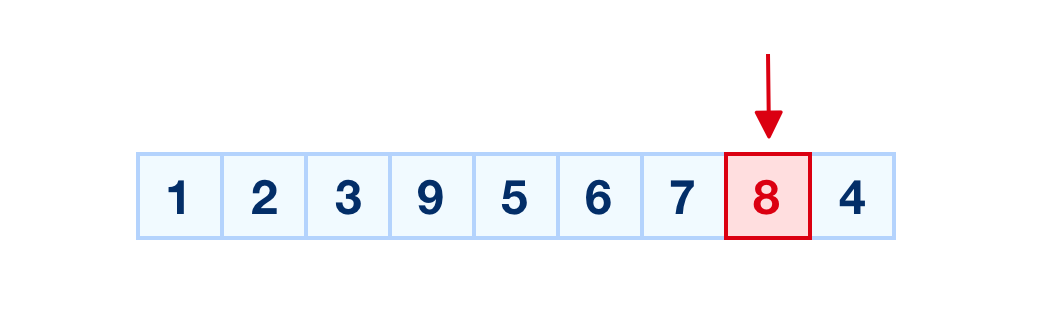Similar to before, it is time for us to pick another item from our array to swap with our selected item. The range of numbers we can pick from is the following: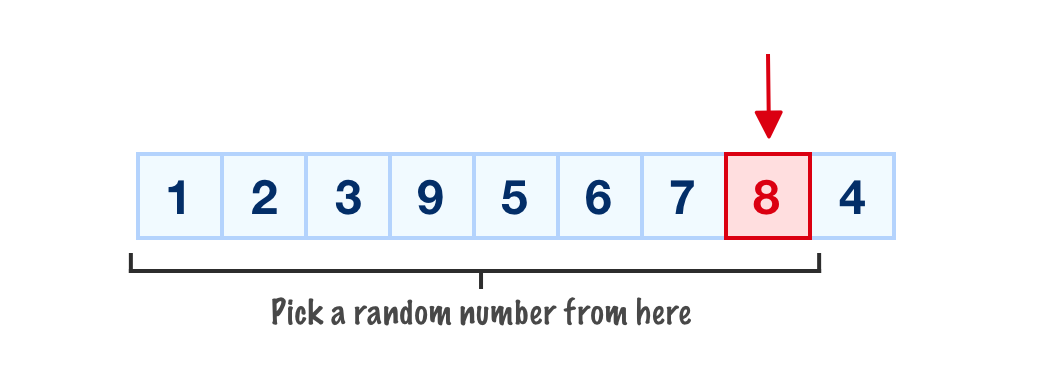Notice that the last item is no longer eligible for being swapped. The items we can pick, to reiterate what we mentioned earlier, are the selected item and all the items that precede it. For our example, the random number we'll pick will be the 2: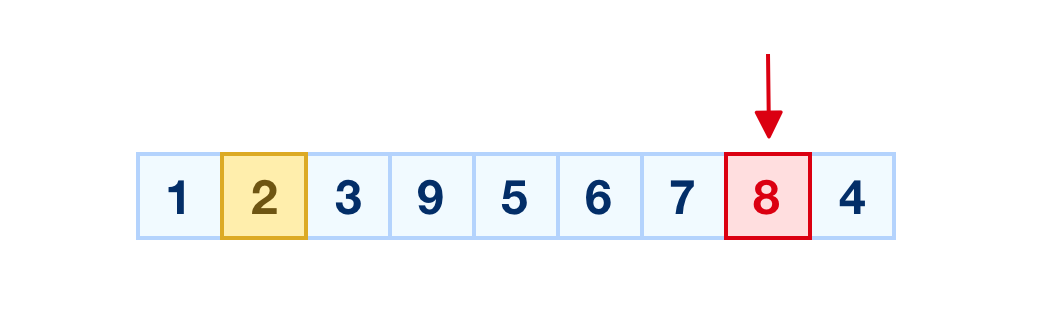Once the random item is picked, we perform the swap with our selected item: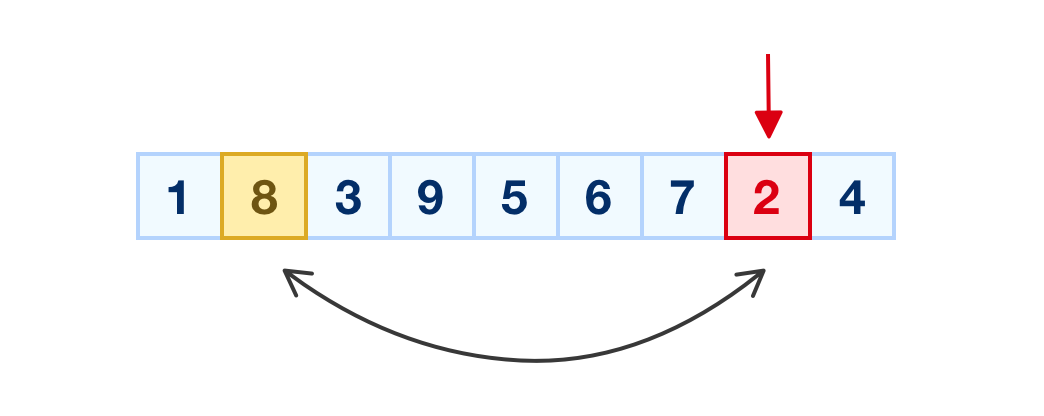After the swap has been done, this whole process continues all over again by us moving on to the next previous item and repeating everything we've seen so far. Now, I could go on explaining our shuffle approach with each remaining item, but that will probably be really boring for you. Instead, the two cases we looked at should prepare you well for playing out the remainder of the items. Our approach for shuffling elements stops when we reach the end of our array, which is actually the first item since we are traversing our array backwards.

Now, let's shift gears to go from words and images to code and see how everything fits together nicely.

## Looking at the Code

In the preceding section, we got an overview of how our algorithm works. Let's now look at how all of that translates into code...starting with the shuffle function:

``````Array.prototype.shuffle = function() {
let input = this;

for (let i = input.length-1; i >= 0; i--) {

let randomIndex = Math.floor(Math.random() * (i + 1));
let itemAtIndex = input[randomIndex];

input[randomIndex] = input[i];
input[i] = itemAtIndex;
}
return input;
}		``````

The shuffle function hangs off an Array object. The way we reference the array and its contents from inside this function is via the this keyword:

``let input = this;``

In our example, it is the input variable that is the lucky one that stores a reference to our array.

The next thing we will look at is the for loop:

``````for (let i = input.length-1; i >= 0; i--) {

let randomIndex = Math.floor(Math.random() * (i + 1));
let itemAtIndex = input[randomIndex];

input[randomIndex] = input[i];
input[i] = itemAtIndex;
}``````

This loop is responsible for going through every item in our array and swapping it with a random number. Notice that the direction of this loop is backwards. We start at the end of our array (input.length - 1) and stop at the beginning. The current position we are in the loop is defined by the i variable, which we referred to as the selected item from our walkthrough earlier:The next step is for us to pick our random number:

``````let randomIndex = Math.floor(Math.random() * (i + 1));
let itemAtIndex = input[randomIndex]; ``````

The randomIndex variable stores the random number mapping to an item's position that we are interested in. Notice that the maximum value of our random number is not the array's length. It is the current index position, for this our design where the random number we can pick is either our selected element (i) or anything that precedes it:Once we have our random item position, we can get that item's contents by directly referring to it:

``let itemAtIndex = input[randomIndex]; ``

At this point, your code corresponds to the following diagram:The red arrow corresponds to the position defined by i, and the item colored in yellow is our randomly selected item (itemAtIndex).

Once we have your randomly selected item, all that is left to do is swap their values:

``````input[randomIndex] = input[i];
input[i] = itemAtIndex;``````

The swapping is done by the above two lines where we first set the item at position i as the item our randomIndex position is pointing to. At this very moment, the contents of input[i] can be found both at the i position but also at the randomIndex position. This is only a temporary situation though.

Earlier, we created a copy of the contents at input[randomIndex] in our itemAtIndex variable. This means we can rectify this problem by setting this copied value back to input[i], which is what the last line highlights.

## Conclusion

Wohoo! You just finished learning how to shuffle the contents of an array. While our example focused on a simple array containing just numbers as values, our array's contents can be anything. Numbers were just easier to visualize and track, so don't feel like you have to only use numbers to take advantage of the shuffling approach shown here. The world of shuffling algorithms is quite interesting because there are many ways to shuffle the contents of an array. A lot of people have done a lot of work over many decades documenting many of those ways. The Fisher, Yates, and Knuth approach described here is just one of the more popular (and efficient) ways.

Got a question or just want to chat? Comment below or drop by our forums (they are actually the same thing!) where a bunch of the friendliest people you'll ever run into will be happy to help you out!